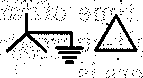# Two power plants interconnected by a tie-line as shown in the figure below have loss formula coefficient B11 = 10-3 MW-1 Power is being dispatched economically with plant `1' as 100 MW and plant '2' as 125 MW. The penalty factors for plants 1 and 2 are respectively

1.  1 and 1.25

2.  1.25 and 1

3.  1 and zero

4.  zero and 1

4

1.25 and 1

Explanation :
No Explanation available for this question

# The below diagram shows the layout of a power station having two generators A and B, connected to the 11kV buses which are also fed through two transformers C and D from a 132kV grid. The 11kV buses are interconnected through a reactor R The reactances of A, B, C, D and R are in p.u. on a common MVA- and kV-base. All the generated voltages in A, B and grid are each 1.0 p.u. a n d assumed as in phase at the time of fault. The steady state symmetrical fault-current for a 3-phase fault on the 11 kV feeders is

1.  10 p.u.

2.  15 p.u.

3.  20 p.u.

4.  25 p.u.

4

15 p.u.

Explanation :
No Explanation available for this question

# The reflection coefficient for the transmission line shown in figure at point P is

1.  + 1

2.  0.5

3.  0

4.  -1

4

0

Explanation :
No Explanation available for this question

# The electrical stiffness of asynchronous generator connected to a very large grid can be increased by

1.  increasing the excitation or the power angle of the machine

2.  reducing the excitation or the synchronous reactance of the machine

3.  increasing the synchronous reactance of the machine

4.  operating the generator at a much lower MW level compared to the steady-state limit

4

increasing the synchronous reactance of the machine

Explanation :
No Explanation available for this question

# The above circuit shows a 3-phase system having a balanced self-inductive reactance of XL per phase and a mutual reactance of X, between pairs of phases. The line currents are Ia, Ib and Ic, and they may be unbalanced The series voltage-drop relations would be Vaa'=IaXL+IbXm+IcXm Vbb'=IaXm+IbXL+IcXm Vcc'=IaXm+IbXm+IcXL The positive, negative and zero-sequence reactances X1, X2 and Xo, respectively, would be

1.  (XL-Xm),(XL-Xm)and(XL-2Xm)

2.  (XL+Xm),(XL-2Xm)and(XL+2Xm)

3.  (XL-2Xm),(XL+Xm)and(XL+Xm)

4.  (XL+2Xm),(XL+2Xm)and(XL+Xm)

4

(XL-Xm),(XL-Xm)and(XL-2Xm)

Explanation :
No Explanation available for this question

# Consider a power system with two plants Si and S2 connected through a tie line as shown above. When the load-frequency control of the system is considered, the 'Flat tie-line control' system is preferred over the 'Flat frequency regulation system', because

1.  it is advantageous to control the frequency from any one particular plant without disturbing the other one during load-swings on either Si. or S2 areas

2.  this ensures that only the more efficient plant's input is controlled for load variation in any area

3.  only the tie line is required to absorb the load-swing

4.  the load-change in a particular area is taken care of by the generator in that area resulting in the tie-line loading to remain constant

4

this ensures that only the more efficient plant's input is controlled for load variation in any area

Explanation :
No Explanation available for this question

# The combined effect of series and shunt compensation, on transmission lines in terms of degree of series compensation (Kse), degree of shunt compensation (Ksh), and surge impedance of uncompensated line (Z0) is given by which one of the following equations x

1.  Z'0=Z0√1-Kse.√1-Ksh

2.  Z'0=[√1-Kse.√1-Ksh]/Z0

3.  Z'0=√(1-Kse)/(1-Ksh)

4.  Z'0=Z0√(1-Ksh)/(1-Kse)

4

Z'0=√(1-Kse)/(1-Ksh)

Explanation :
No Explanation available for this question

# If a sudden short circuit occurs on a power system (considered as R-L series circuit), the current waveform consists of 1. a decaying a.c. current. 2. a decaying d.c. current. Let the alternator reactance be X and the power system resistance R. Which one of the following is correct

1.  The decay in (1) is caused by the increase in but in (2) is caused by R

2.  The decay in (1) is caused by R caused by increase in X

3.  The decay in both (1) and (2) is ca-used by R

4.  The decay in both (1) and (2) is caused by the increase in X

4

The decay in (1) is caused by the increase in but in (2) is caused by R

Explanation :
No Explanation available for this question

# A zero-sequence network of a 3-phase transformer is given below. Zo is the zero-sequence impedance of the transformer. What is the type of transformer connections

1.

2.

3.

4.

4Explanation :
No Explanation available for this question

1.  168.75W

2.  112.5W

3.  75W

4.  50W

4